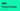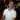# VBA Functions

May 28, 2021

A VBA Function is like a Sub Procedure where it could be thought of as a container for your VBA code. The difference is that VBA Functions can return a value back to the caller* where a Sub Procedure cannot return a value. With this in mind, the kind of code you want inside of functions would be the kind where you need to take some inputs, calculate a value, and return that value.

* A caller is some other Sub Procedure or Function (or even Worksheet cell) that invoked the VBA Function.

Where functions really shine is that you can use them inside an Excel formula.

## How to Create Functions

Here’s an example to get us started. Create a new module and place this code inside:

``````Option Explicit

Function DoubleNumber(ByVal number As Long) As Long
DoubleNumber = number * 2
End Function``````

Here, we have a function called `DoubleNumber` which doubles any number passed into it. The way that we return a value back to the caller is by using this convention:

``MyFunctionName = SomeValue``

Which is why you see the function name `DoubleNumber` being assigned a value. This is much different from other languages that might use the `return` keyword. You can also set the function’s return value several times before the function ends:

``````Option Explicit

Function DoubleNumber(ByVal number As Long) As Long
DoubleNumber = 0
DoubleNumber = number * 2
End Function``````

While this is perfectly legal to do, I would recommend you only set the function’s return value at the very end of the function so your code is more readable.

The data type you return is defined at the end of the `Function` declaration, as shown by the ending `As Long` statement here:

``Function DoubleNumber(ByVal number As Long) As Long``

If you didn’t include that `As Long` part at the end, the return type would default to `Variant`. I highly recommend always returning a specific return type if you can.

You can also exit the function early with the `Exit Function` command.

``````Option Explicit

Function DoubleNumber(ByVal number As Long) As Long
' Only double positive numbers
If (number < 0) Then
Exit Function
End If

DoubleNumber = number * 2
End Function``````

This also shows that you don’t have to set a return value for the function if you don’t want to. If `number` is a negative number, the function looks like it returns nothing, but it actually returns the default value for `Long` which is zero.## Where to Use Functions

You can use functions from within other VBA code or you can use them from your Worksheet. This lets you create your own custom Excel worksheet functions which are called User Defined Functions.

After adding that `DoubleNumber` function into your module, you can apply it into cell `A1`:

``=DoubleNumber(2)``

Which returns `4` and places that into the cell.

You will also see the function name appear in the help popup when typing the formula:

If you don’t want these functions to show up in the Excel worksheet formula helper, you can mark the function `Private`:

``````Option Explicit

Private Function DoubleNumber(ByVal number As Long) As Long
If (number < 0) Then
Exit Function
End If

DoubleNumber = number * 2
End Function``````

Note that this only hides the function from the popup, but it’s still available to use from the worksheet if you happen to know the name.

Also, if you place a VBA Function inside Worksheet / Workbook / Userform code and not in a Module, then the function will not be available to any worksheet cells, but only VBA code that has visibility to that function (even if you mark the function `Public`).

## When to Use Functions

If you need to calculate data in a custom way, definitely consider using a Function over a Sub Procedure. People are more accustomed to writing formulas in Excel than they are with running macros, so you’ll lower the barrier to adoption if you can use functions.

If you’re looking to keep your VBA code clean, functions can help you manage code complexity and keep your code readable. Take this function that switches the name order for example:

``````Public Sub ConvertNames()
Dim myStrings As Excel.Range
Set myStrings = Application.Selection

Dim names() As String
Dim namesFormatted() As String
Dim myString As Excel.Range
Dim i As Long

For Each myString In myStrings
ReDim Preserve namesFormatted(i)

names = Split(myString, ",")
names(0) = Application.WorksheetFunction.Trim(names(0))
names(1) = Application.WorksheetFunction.Trim(names(1))

namesFormatted(i) = names(1) & " " & names(0)
i = i + 1
Next

For i = 0 To UBound(namesFormatted)
Debug.Print (namesFormatted(i))
Next
End Sub``````

If you have two names in cells `A1:A2` like this:

``````Smith, Agent
Anderson, Thomas``````

When you select this range and run the code, the VBE Immediate Window will print out the following:

``````Agent Smith
Thomas Anderson``````

While this is a small example, the logic that splits the names, removes extra spaces, and reverses the order could be more clear. This is a good use case for using a function to take care of that logic.

Sidenote: any time you see a loop with logic like this in it, consider moving that logic to its own function. It can help make the code more readable and you can reuse that logic in other parts of the code.

Let’s refactor this code by having the logic that switches the names around be in its own function:

``````Public Sub ConvertNamesRefactored()
Dim myStrings As Excel.Range
Set myStrings = Application.Selection

Dim namesFormatted() As String
Dim myString As Excel.Range
Dim i As Long

For Each myString In myStrings
ReDim Preserve namesFormatted(i)

namesFormatted(i) = SwitchNameOrder(myString.Value)
i = i + 1
Next

For i = 0 To UBound(namesFormatted)
Debug.Print (namesFormatted(i))
Next
End Sub

Private Function SwitchNameOrder(ByRef lastNameFirst As String) As String
Dim names() As String
names = Split(lastNameFirst, ",")
names(0) = Application.WorksheetFunction.Trim(names(0))
names(1) = Application.WorksheetFunction.Trim(names(1))

SwitchNameOrder = names(1) & " " & names(0)
End Function``````

Here, we’ve made a function to handle swapping the name order, where the sub procedure handles dealing with the worksheet code and printing the names. This also helps with separating concerns in your code.

Now when you read that line:

``namesFormatted(i) = SwitchNameOrder(myString.Value)``

You’ve given the logic a name, `SwitchNameOrder`, which makes the code a lot more readable. And believe me, spending the time to make your code more readable can save you a headache or two. Especially when you don’t touch your code for months and you have to remember what you did and why.

Wow, you read the whole article! You know, people who make it this far are true learners. And clearly, you value learning. Would you like to learn more about Excel? Please consider supporting me by buying me a coffee (it takes a lot of coffee to write these articles!).Written by Joseph who loves teaching about Excel.